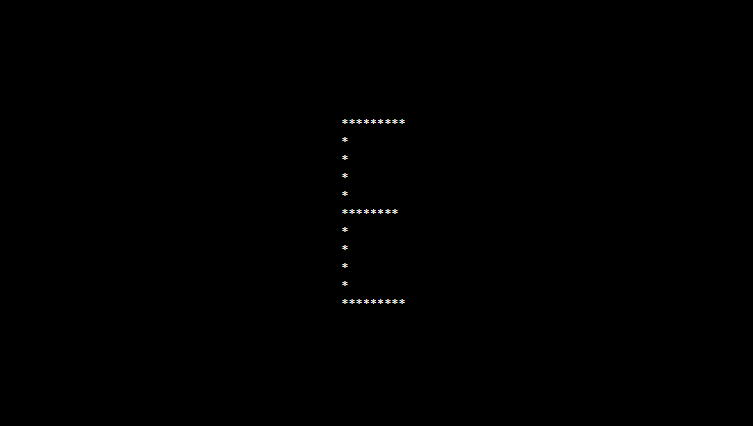# PHP program to print alphabet pattern E

In this article write a PHP program to print alphabet pattern E. This Program first takes the numbers of rows and then prints pattern using nested for loops.

## PHP program to print alphabet pattern E

```<?php
echo "<pre>";
for (\$row = 0; \$row < 11; \$row++) {
for (\$col = 0; \$col <= 11; \$col++) {
if (\$col == 1 or ((\$row == 0 or \$row == 10) and (\$col > 1 and \$col < 10)) or (\$row == 5 and \$col > 1 and \$col < 9)) {
echo "*";
} else {
echo "&nbsp;";
}
}
echo "<br/>";
}
echo "</pre>";
?>
```

### Output:1. Raj says

if i want this in small case like e then which pattern i should do help me!!

1. Full Stack developer says

try this code –

```<?php
echo "<pre>";
for (\$row = 0; \$row < 11; \$row++) {
for (\$col = 0; \$col <= 11; \$col++) {
if ((\$col == 1 AND \$row != 0 AND \$row != 10) OR ((\$row == 0 OR \$row == 10) AND \$col > 1 AND \$col < 9) OR (\$row == 4 AND \$col > 2 AND \$col < 10) OR (\$col == 9 AND \$row != 0 AND \$row != 5 AND \$row != 6 AND \$row != 7 AND \$row != 8 AND \$row != 10)) {
echo "*";
} else {
echo " ";
}
}
echo "<br/>";
}
echo "</pre>";
?>
```